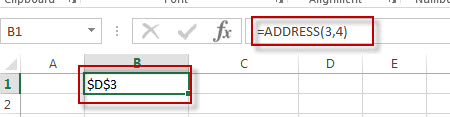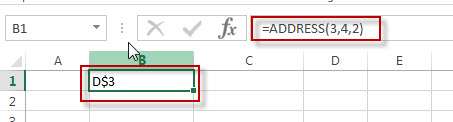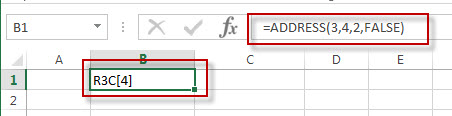This post will guide you how to use Excel ADDRESS function with syntax and examples in Microsoft excel.

### Description

The Excel ADDRESS function returns a reference as a text string to a single cell.

The ADDRESS function is a build-in function in Microsoft Excel and it is categorized as a Lookup and Reference Function.

The ADDRESS function is available in Excel 2016, Excel 2013, Excel 2010, Excel 2007, Excel 2003, Excel XP, Excel 2000, Excel 2011 for Mac.

### Syntax

The syntax of the ADDRESS function is as below:

`=ADDRESS (row_num, column_num, [abs_num], [a1], [sheet_text])`

Where the ADDRESS function arguments are:

• row_num -This is a required argument. The row number to use in the cell reference.
• column_num – This is a required argument. The column number to use in the cell reference.
• Abs_num – This is an optional argument. It will specify the type of reference to use. The following values can be used:

1 – Absolute

2 – Absolute row; relative column

3 – Relative row; absolute column

4 – Relative

A1 – This is an optional argument. It will specify the style of reference to use.

• True– A1 reference style
• False – R1C1 reference style

Sheet_text – This is a required argument. The sheet name to use.

### Example

The below examples will show you how to use Excel ADDRESS Lookup and Reference Function to return a reference as a text.

#1 Absolute reference, type formula: =ADDRESS(3,4)#2 Absolute row, relative column, type formula: =ADDRESS(3,4,2)#3 Absolute row, relative column in R1C1 reference style, type formula:=ADDRESS(3,4,2,FALSE)